# ROTAMASS 3 Series Coriolis Mass Flow and Density Meter

Shuuji Urabe1 Kiyoaki Koyama1

The Yokogawa Group has developed the ROTAMASS 3 series Coriolis flow and density meters. A Coriolis flow meter can directly measure mass flow of fluid regardless of its temperature and pressure. The ROTAMASS 3 series meters have such unique features as fast signal processing, anti-vibration mechanism, and high accuracy density measurement. This paper describes these features and applications of the ROTAMASS 3 series.

### INTRODUCTION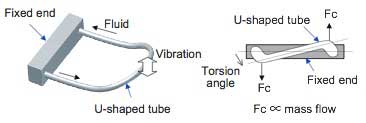Figure 1 Measurement Principle of the Coriolis Flow Meter (1)

The Coriolis flow meter has many features such as direct measurement of mass flow and measurement of fluid density, which other flow meters do not support. Therefore, since being introduced for practical use in the late 1970s, its market has rapidly expanded in many industries such as oil, chemicals, and foods. The YOKOGAWA Group has also been focusing interest on its features and continued R&D in this area. Eventually, following joint R&D with ROTA YOKOGAWA GmbH & Co. KG (Germany), Yokogawa released the ROTAMASS series Coriolis flow meters in 1993 worldwide, mainly for the Europe, North America, and Southeast Asia markets.

Process flow meters must be able to measure flow rates at high speed and accuracy, and must also be robust to withstand stresses and vibrations applied through the process connection with the piping. To achieve these properties, we developed a high speed high accuracy digital signal processing algorithm and a Box-in-Box structure to reduce the impact from external force and vibration. This paper describes the features of the signal processing and structure, and application examples of the ROTAMASS series Coriolis mass flow and density meters.

### MEASUREMENT PRINCIPLE AND FEATURES OF THE CORIOLIS FLOW METER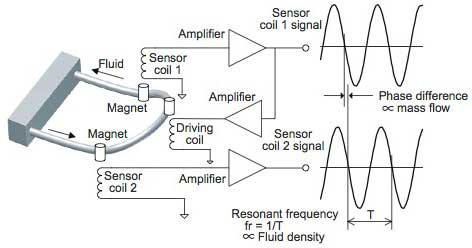Figure 2 Measurement Principle of the Coriolis Flow Meter (2)

A Coriolis mass flow meter measures the mass flow of fluid based on the interaction of Coriolis forces generated between the flowing fluid and vibrating measurement tube. The principle is outlined in Figure 1. The U-shape tube is repeatedly swung around the fixed end as a pivot like a cantilever. When fluid flows in this tube, Coriolis forces (Fc) are generated at both the inlet and outlet sides of the tube according to the ascent and descent of the tube. Since the directions of forces generated at inlet and outlet sides are opposite, torque is created generating torsion angle of the tube. Because the Coriolis force Fc is proportional to the mass flow of the fluid, the mass flow can be measured by detecting the torsion angle.

Figure 2 shows the flow meter with the major elements necessary for measurements and signals. A pair of a magnet and driver coil is mounted at the apex of the U-shaped tube, and two pairs of a magnet and sensor coil are arranged at the upstream and downstream sides respectively. The positive feedback of electromagnetically induced output of the sensor coil to the driver coil leads to self-oscillation of the measurement tube at its resonant frequency. The torsion angle of the tube can be calculated based on the phase difference between the output signals of the two sensor coils. That is, the mass flow rate can be derived from the phase difference of the two signals. It is possible to calculate simultaneously the mass of the fluid based on the signal frequency, from which the density can be derived.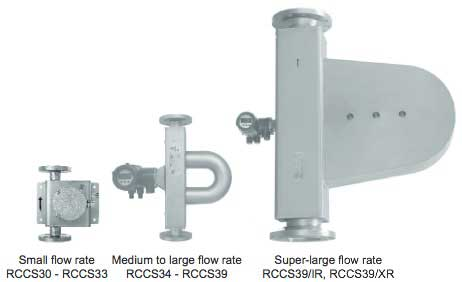Figure 3 External View of ROTAMASS 3 Series Flow Meters

A Coriolis mass flow meter:

1. can directly measure the mass flow rate based on the principle of measurement,
2. can measure the mass flow rate with high accuracy of ±0.1%,
3. has a wide measurement flow rate range,
4. can measure the density of fluid based on the oscillating frequency,
5. is not affected by viscosity and density of the fluid, and does not require straight pipe sections upstream nor downstream of the flow meter, and
6. can measure non-conductive fluids.

In general, chemical reactions, etc. are described in moles or masses of substances. Therefore, it is very useful to be able to directly measure the mass flow rate in chemical and pharmaceutical industry applications. Other flow meters such as differential pressure, electromagnetic, and vortex flow meters must convert from volume flow rate to mass flow rate while compensating for temperature and pressure. Although magnetic flow meters can measure only electro-conductive fluids due to the principle of measurement, Coriolis mass flow meters can measure non-conductive fluids. This is one reason why they are widely used in the chemical and pharmaceutical industries

### SPECIFICATIONS

Yokogawa's Coriolis flow meter started with the ROTAMASS 1 series released in 1993. Since then, converters were improved in the ROTAMASS 2 series, and advanced technologies were introduced in both detectors and converters in the current ROTAMASS 3 series. This paper focuses on the ROTAMASS 3 series flow meters currently in the market.

Figure 3 shows the external view, and Table 1 shows the major specifications including the flow rate range and measurement accuracy. As the external view shows, the ROTAMASS series flow meters have a U-shaped measurement tube. The flow rate range covers from less than 0.1 t/h to 500 t/h at the nominal flow rate (Qnom) and pressure drop of 0.1 MPa.

Table 1 Major Specifications of ROTAMASS 3 Flow Meters

Model Nominal flow rate Qnom [t/h] Maximum flow rate Qmax [t/h] Accuracy of mass flow rate measurement [% of flow rate] Zero stability [kg/h] Accuracy of density measurement [g/cm3]
Standard Option /K6
Small flow rate RCCS30 0.045 0.1 ± 0.1 ± Zero stability/ flow rate * 100 0.05 0.008 -
RCCS31 0.17 0.3 0.0085 0.004 -
RCCS32 0.37 0.6 0.019 0.004 0.0005
RCCS33 0.9 1.5 0.045 0.004 0.0005
Medium to large flow rate RCCS34 2.7 5 0.135 0.003 0.0005
RCCS36 10 17 0.5 0.0022 0.0005
RCCS38 32 50 1.6 0.0015 0.0005
RCCS39 100 170 5 0.0015 0.0005
Super-large flow rate RCCS39/IR 250 300 13 0.0015 -
RCCS39/XR 500 600 25 0.0015 -

### FEATURES OF ROTAMASS 3 SERIES FLOW METERS

High-speed Signal Processing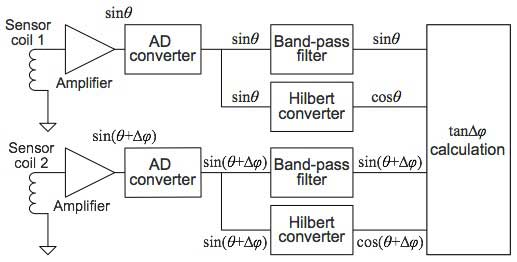Figure 4 Signal Processing in the ROTAMASS

This section describes the signal processing of the ROTAMASS. As noted in the section regarding the measurement principle, the mass flow can be calculated based on the phase difference of two sensor coil signals. In the early models, the mass flow was calculated based on the time difference of the zero cross point of the sensor coil signals. However, this method was susceptible to noise superimposed on the signals. Thus, as technologies for analog-to-digital converters (ADC) and digital signal processors (DSP) advances, the procedure where all signals are digitized and the phase difference is calculated by a digital Fourier transform by DSP is mostly applied. Yet with this method, the wavelength interval of the tube vibration must be equally divided and sampling at those points is required, resulting in a complex sampling timing generation circuit. Moreover, it has the disadvantage in which the output cannot follow the fluctuation of the density and temperature of the fluid to be measured, because the vibrational frequency of the tube fluctuates depending on the density and temperature.

To solve these problems, we invented a signal processing method to calculate the phase angle by using the Hilbert transform for the ROTAMASS (Japan Patent No. 3219122 and United States Patent No. 5578764). Figure 4 shows a schematic block diagram of the signal processing. The signals of sensor coil 1 and sensor coil 2 are assumed to be sinθ and sin (θ + Δφ), respectively. The Hilbert converter is a digital filter to shift the phase of the input signal by 90 degrees and output it. When the input signal is sinθ , the output signal of the Hilbert converter is cosθ. By using the outputs of each digital filter, the phase difference Δφ can be calculated by the following equation.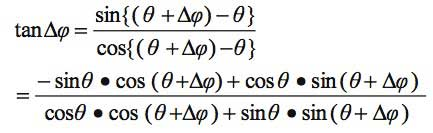This signal processing method has the following features.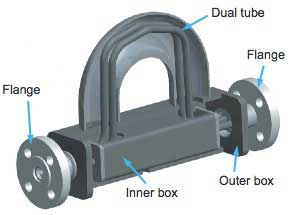Figure 5 Structure of the ROTAMASS Detector
1. Signals can be sampled at a fixed sampling frequency independent of the vibrational frequency. Therefore, no complex timing generation circuit is required to follow the vibrational frequency that fluctuates depending on the density, temperature, etc. of the target fluid. In addition, accurate outputs can be obtained with a simple configuration using the inexpensive, accurate ΔΣ type AD converter.
2. Phase difference signals can be obtained at every sampling point, achieving high-speed mass flow rate outputs.
3. The amount of calculation required in the DSP is about one-quarter that of the conventional FFT calculation method; the load for the calculation is light.

Vibration Resistance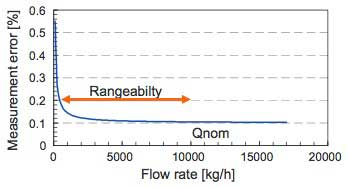Figure 6 Accuracy of Mass Flow Rate

Coriolis flow meters measure flow rates by vibrating the measurement tubes like a tuning fork. Therefore, the measurements may be affected by external forces or vibrations transmitted through pipes. The ROTAMASS series has an original structure called "Box-in-Box" which effectively eliminates the effects of external forces and vibrations.

Figure 5 shows the detector structure of the ROTAMASS. The inner box is mounted in the outer box which is connected to the flanges. Both ends of the tubes are fixed to the inner box. This double structure enables external forces and vibrations applied through the flanges to be absorbed by the distortion of the structure such as the outer box, minimizing the deformation of the tubes fixed to the inner box.

In addition, a two-tube structure is employed, and they are vibrated in opposite directions. The sensor coils for measuring the vibration are mounted on one tube while the magnet is attached to the other tube. Owing to this structure, the tubes are not easily affected by external vibration. Moreover, tuning the resonant frequency of the tubes considerably higher than the expected vibrational frequency of disturbances in the field minimizes the impact of the external vibration.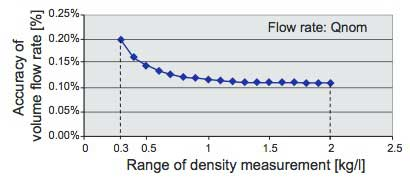Figure 7 Volume Flow Rate Measurement Accuracy with High-accuracy Density Measurement

Another structural feature is the angle given at the linear part of the U-shaped tubes. This enables the fluid in the tubes to drain by itself.

High Rangeability

Generally, Coriolis flow meters have wider rangeability (measurable flow range: the ratio of the measurable maximum flow rate to the minimum flow rate) than other flow meters. The practical rangeability of Coriolis flow meters depends on the error of the zero stability which represents fluctuation at the zero point. The error of zero stability of the ROTAMASS 3 series is small, and so it maintains excellent measurement accuracy even at low flow rates. Figure 6 shows a diagram of the flow rate and the mass flow measurement accuracy. Rangeability of 20 is secured assuming that the measurable maximum flow rate is the nominal flow rate (Qnom) and the necessary accuracy is 0.2%.

Highly Accurate Density Measurement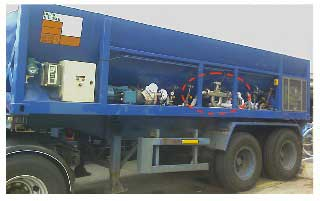Figure 8 Application Example in a Road Tanker

Improving the density measurement accuracy also enhances the accuracy of volume flow rate and concentration measurements because they are obtained as a function of density.

The standard specifications for the density measurement accuracy of the ROTAMASS 3 series is 0.008-0.0015 g/cm3 (Table 1). By selecting the option /K6, even more accurate measurement of 0.0005 g/cm3 is available. To achieve this accuracy, we have developed a special calibration device that uses three types of liquid. Every flow meter guarantees the accuracy of a wide range of 0.3-2.0 g/cm3 by calculating the correction factor based on the fluid the temperature of which is controlled. Figure 7 shows the accuracy of volume flow rate measurement with the high-accuracy density measurement option of 0.0005 g/cm3. This figure shows clearly that the volume flow rate can be measured very accurately by using this option.

### APPLICATION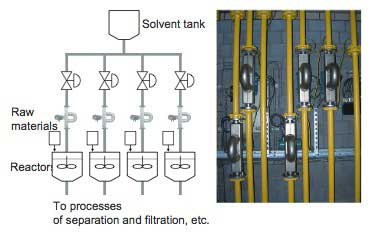Figure 9 Application to a Pharmaceutical Process

This section describes some applications of the ROTAMASS 3 series. Figure 8 shows an example of installation in a track for road tanker for liquefied gas utilizing the high resistance to vibration of the Box-in-Box configuration. This flow meter has been performing stable measurements for more than ten years even in this environment with severe vibration.

High rangeability and high-precision measurement at low flow rate are extremely effective features in the chemical and pharmaceutical industries. Figure 9 shows an example in the pharmaceutical industry. In this process, some solvents are supplied to multiple reactors. Coriolis flow meters with high-precision measurements were adopted because it is crucial to measure the flow rate accurately.

### CONCLUSION

This paper described the principle and features of the Coriolis flow meter and the features of Yokogawa's ROTAMASS series Coriolis flow meter and its applications. The Coriolis flow meter is based on the principle discovered by Coriolis in the 19th century. However, the measurement technology applying this principle is rather new, because the Coriolis flow meter only started to develop rapidly since the 1990s, during which signal processing technologies using AD converters, CPUs, and DSPs progressed remarkably.

Applications of the Coriolis flow meter are expected to expand as an excellent mass flow meter. Users' demands vary with industry and region: larger diameter for the oil and gas industries, low flow rate for batch processes in fine chemicals and pharmaceuticals, and low price for Southeast Asia. The ROTAMASS series have been used in various industries since their release and have been steadily improved in accordance with users' need for applications. We will continue to meet such needs through constant enhancements; improving accuracy, lowering price, expanding interfaces with upper systems and so on.

### 相关产品&解决方案

#### 流量计